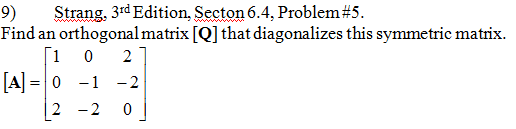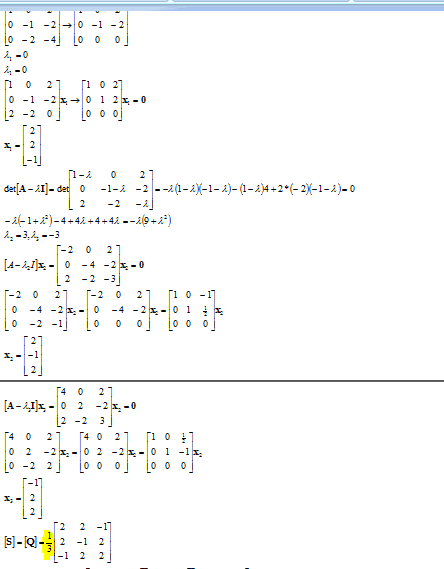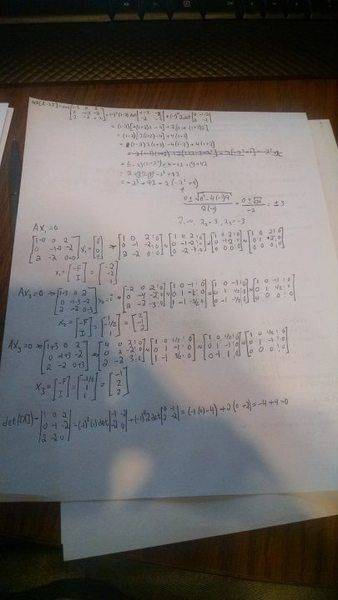# Linear Algebra - Find Orthogonal Matrix Q that diagonals

YoshiMoshi

## Homework Statement

I'm told to find the matrix Q of the matrix A## The Attempt at a Solution

So my problem is that in the answer key they have S = (1/3)... and I have no idea where this 1/3 comes from. I get an equivalent answer for X_1, X_2, and X_3
S = [X_1, X_2, X_3] but when I form this matrix with the values for X_1, X_2, X_3 I'm not able to factor out a 1/3. Does anyone know where this value comes from?
This is the solutionsand this is my attempt, again I would get the same answer but have no idea were the 1/3 came from.thanks for any help

Homework Helper
An orthogonal matrix is a unitary matrix. Therefore ##Q^*Q = QQ^*=I##. This is why the vectors making up the column of ##Q## must be orthonormal - 1/3 is a normalization constant.

YoshiMoshi
How do I calculate this normalization constant? Thanks for your help. Like I'm exactly sure which vector I'm normalizing and getting 1/3. But I know to normalize a vector it's

V/||V||

Homework Helper
A vector is said to be normalized if its norm equal unity.

YoshiMoshi
So it's just

1/sqrt((1+1+1)^2)?

Homework Helper
1/sqrt((1+1+1)^2)?
I don't understand what you want to say there.
Suppose you have three component vectors ##u = (a,b,c)^T##. In order to make it normalized you have to add a common constant by yourself to the vector: ##u = \gamma (a,b,c)^T##. By requiring its norm to be equal to one, namely ##\gamma^2(|a|^2 + |b|^2 + |c|^2) = 1##. This way you can find ##\gamma## in terms of the components of ##u##. For a general unitary matrix, ##\gamma## can be complex but since here the problem asks for an orthogonal matrix, ##\gamma## must be real.

YoshiMoshi
Oh I see that makes more since to me know. So it's for the whole Matrix S? When I square all the terms in S and sum each row individually I get 9, so the constant must be 1/sqrt(9) = 1/3.

What if each row doesn't sum up to the same value like it did in this case?